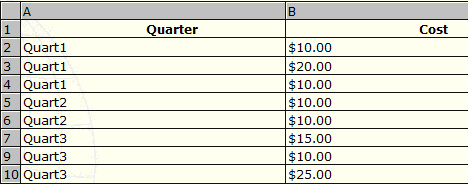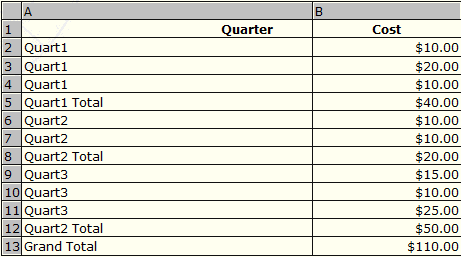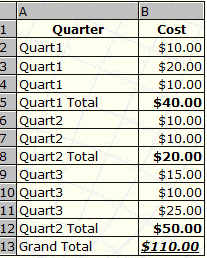Connect with us

# Excel Tips: How to Bold Subtotals AutomaticallyPublished

onOne issue that is often encountered when accountants work in Excel is that the Subtotal results, via Data>Subtotals are not bolded or made easily distinguishable. This can make the resulting data very hard to read, especially if the table that we have applied Subtotals to contain many columns. This often means the resulting subtotals are over to the right, while their associated headings are often in the first column.

As I said on my status in facebook, I am a bit bored. So let me show you some little tricks how to bold excel sub-total automatically. Even further; how to style the grand total automatically too. Let’s go!

Consider the small example below where Subtotals have been added to a very small table of data.

Before Subtotals:After Subtotals:In the above table our Subtotal headings have been bolded by Excel, yet their associated results have not.  As this table only has two columns, it is not that hard to read and pick out the Subtotal amounts. The more columns that a table has, the harder it becomes to visually pick-out the Subtotals.

### Steps to Automatically Bold the Sub-total

The solution to the above problem is to make use of Excels Conditional Formatting. Using the above table as an example try the following steps before adding your Subtotals:

• Select A1:B10, ensuring the A1 is the active cell.
• Go to Format>Conditional Formatting
• Select Formula is: and then add this formula: =RIGHT(\$A1,5)=”Total”
• Now click the Format button and then the Font tab and then select Bold from Font Style:
• Now click OK, then OK again

The all important part to the formula is the Absolute of the Column (\$A) and the Relative reference of the Row (1). As we Start ed our selection from A1, Excel will automatically change the formula for each cell. For example, cell A2 will have a Conditional Format formula of: =RIGHT(\$A2,5)=”Total”, cell B2 will also have =RIGHT(\$A2,5)=”Total” and cell A3 and B3 will have: =RIGHT(\$A3,5)=”Total”.Note: When you remove the Subtotals, the bolded font will no longer apply.

### Taking It One Step Further; Style the Grand Total

The only problem with the above table now is the Grand Total is bolded the same as the Subtotals. For Grand Totals I prefer to see these formatted different again, in this example I make it italic and Underlined. Here is how we can do this. Again the steps are based on the above examples.

• Select A1:B10, ensuring the A1 is the active cell.
• Go to Format>Conditional Formatting
• Select Formula is: and then add this formula: =\$A1=”Grand Total”
• Now click the Format button and then the Font tab and then select Bold from Font Style:
• Click Ok, then click Add to add a second Format Condition
• Select Formula is: and then add this formula: =RIGHT(\$A1,5)=”Total”
• Now click the Format button and then the Font tab and then select Bold Italic from Font Style: and then Single from Underline:
• Now click OK, then OK again

Your Subtotals will now look like below:Note: You can of course use any format you like to make your Subtotals easier to read.

Are you looking for easy accounting tutorial? Established since 2007, Accounting-Financial-Tax.com hosts more than 1300 articles (still growing), and has helped millions accounting student, teacher, junior accountants and small business owners, worldwide.